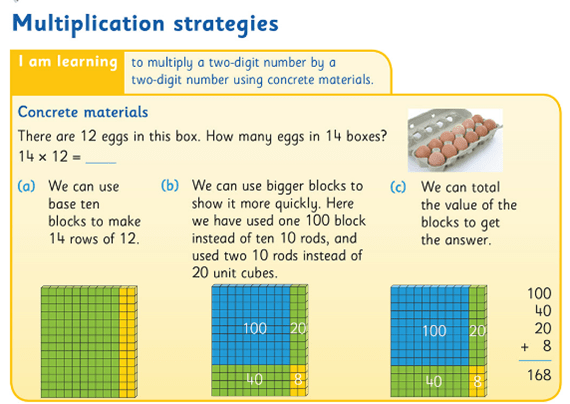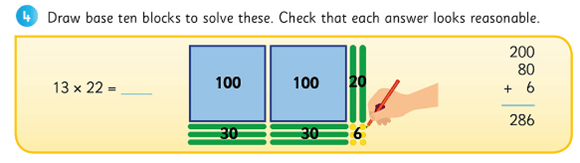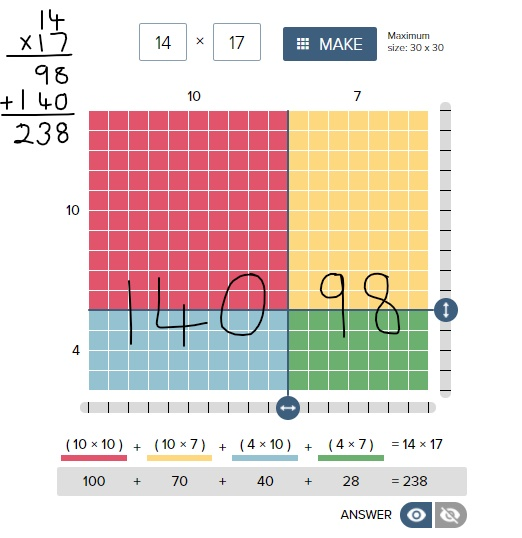# Digging Deeper into … Multiplication and Division (3rd – 6th classes)

## Digging Deeper into … Multiplication and Division (3rd – 6th classes)

Category : Uncategorized

For practical suggestions for families, and links to useful digital resources, to support children learning about the topic of multiplication and division, please check out the following post: Dear Family, your Operation Maths Guide to Multiplication and Division

Children are formally introduced to the operations of multiplication and division in third class, although their initial exploration of these concepts would have begun in 1st and 2nd classes with basic skip counting in jumps of 2s, 10s, 5s and, to a lesser extent, 3s and 4s. Thus, most children become quite comfortable with the number facts of twos, fives and tens quite quickly because these facts are more familiar to them. That said, when starting multiplication and division, and particularly when doing these groups of “easier” facts, it is still very important that the children are given ample time and experiences to develop a solid understanding of the concepts of multiplication and division themselves. This is why this topic is typically taught as a double (i.e. two week) chapter in Operation Maths.

Furthermore, as with the Operation Maths approach to addition and subtraction, multiplication and division is, for the most part, taught together, so as to reinforce them as related concepts that are also the inverse of each other. Thus, the initial activities in the Discovery Book, often require the children to reflect on their understanding of the concepts and to compare and contrast them.

As the children move through the classes, it is anticipated that they would begin to use this understanding of multiplication and division as the inverse of each other, to recognise the quotient (answer) in division as a missing factor in the inverse multiplication fact, e.g. asking what is 45 ÷ 9 is the same as asking how many groups of 9 equals 45 or [ ] × 9 = 45. This knowledge will also help as the children move towards more efficient recall of the basic division facts.

### CPA Approach

As always, Operation Maths advocates a concrete–pictorial–abstract (CPA) approach to this topic. In 3rd class, this means the children will be moving from experiences with familiar objects that are already pregrouped (eg three wheels on a tricycle, four wheels on a car, ten toes on a person) and/or groups they create themselves using objects, to pictorial activities (e.g. where the children draw representations of the numbers using  pictures of the concrete materials) and finally to abstract exercises, where the focus is primarily on number sentences and the use of the multiplication and division symbols.Using base ten blocks to demonstrate multiplication as an area array

Similarly, in 4th to 6th classes, the approach taken should start with concrete materials (eg base-ten blocks to represent multiplication using the area model, as shown above) as demonstrated by the images in the Pupil’s books and explained in the relevant Teacher’s Resource Book (TRB). As always, the emphasis is on the children understanding the process before being introduced to the formal algorithms (abstract stage). Otherwise, they are just being taught to use a procedure to produce an answer without really understanding why or how it works.Moving on from the actual blocks; drawing an area model as a pictorial representation of the calculation.

Teachers should use opportunities such as questioning, conferencing etc. to assess their children’s understanding as opposed to just checking for a correct answer.  In particular, check does the child truly understand the purpose of the zero in the second line of the long multiplication algorithm; often when questioned a child will say they need a zero or the answer will be wrong, without appreciating that they are now multiplying by a multiple of ten, so the digits must be moved up a place, hence the necessary zero as placeholder. Therefore, in the example below, 14 is first multiplied by 7, giving a product of 98 on the first line, and then 14 is multiplied by 10 (not 1) giving a product of 140 on the second line. These partial products are then added to give a final total or final product. If in doubt about a child’s understanding, return to the concrete materials and/or pictorial phase again.  Based again on the area model of multiplication, the Partial Product finder tool from the Math Learning Centre can also be very useful to demonstrate this (shown below).If you want even more examples of the effectiveness of using the area model of multiplication, please check out this video from James Tanton. The entire facebook post is accessible here

Similarly, when considering division, while the goal is for the children to be able to divide by two-digit numbers confidently, that doesn’t necessarily have to be via the formal long division algorithm. The long division algorithm has long been a controversial element in primary mathematics. Many feel it is counter-productive to be spending so much time in 5th and 6th class learning and teaching a procedural approach to solve written calculations, when the children will have continual access to a calculator for such calculations once they hit secondary school. In fact, in many other educational systems (UK and various US states included) they have abandoned the teaching of the long division algorithm completely in preference to the ‘chunking method’ (also known as the partial quotients method). However, if the method of the long division algorithm is taught using a CPA approach, as demonstrated in the Operation Maths Pupil’s book for 5th class, it can serve to enhance children’s understanding of number and operations in general.### Language & PropertiesConsistent and accurate use of language is also an important way to emphasise the necessity of this interconnected CPA approach. The way that the number sentences for these operations are verbalised can unwittingly confuse children. If for  2 x 4, we say ‘two multiplied by four’, that implies 2 + 2 + 2 + 2, e.g. four plates with two cookies oneach. However, if we say ‘two times/groups/rows of four’, that implies 4 + 4, e.g. two plates with four cookies on each. While it is understood that the order for multiplication doesn’t matter (i.e. the commutative property of multiplication) and that the answer for both of these is the same, children don’t inherently understand this, especially at the introductory stage. And to further confuse them, when represented concretely or pictorially, both images would also look different.

Therefore, for consistency, initially teachers and students  should use  the language of ‘groups/rows of’ to describe multiplication sentences and use the word times when verbalising the multiplication symbol (×). Similarly, in division we should initially use ‘shared/divided between’ and when using the division symbol (÷) we should say ‘divided into (… equal groups)’. When comfortable with the concept of division as sharing (i.e. 12 ÷ 2 = 6, 12 divided into 2 equal groups is 6 in each group) then the children can be introduced to the concept of division as repeated subtraction (12 ÷ 2 = 6, how many groups of 2 in 12 or how many times can I take  a group of 2 away from 12).

Once the children understand that they can use the commutative property to turn around facts in order to calculate more efficiently, they should be encouraged to recognise how multiplication sentences can be distributed into smaller groups, and that smaller groups can be combined to give the same total i.e  the distributive property of multiplication. It is hoped that they will begin to recognise where these properties can be applied in order to make calculations easier. Encourage the children to read distributed number sentences using the language of ‘groups of’ or ‘rows of’, as this can aid clarity, where the numbers and symbols all together can cause confusion. The 100 dots grids on the inside back covers of Operation Maths 3 and 4 can be extremely useful for the pupils to model various arrangements/arrays, while the teacher can use the 100 square eManipulative to replicate the children’s arrangements on the IWB.Ultimately, being able to understand and apply the properties of multiplication greatly aids fact fluency. Understanding the distributive property, in particular, is key to understanding how the long multiplication algorithm works, i.e. that we multiply by the units and then multiply by the tens and then recombine these partial products. The distributive property and the ability to combine or break apart groups within factors also provides the children with strategies to mentally calculate products and quotients without having to use the standard written algorithms, for example using branching and T-charts, as featured in Operation Maths 5.

### Thinking Strategies for the Basic Number Facts

When most of us were in school, multiplication and division was largely all about tables and learning off tables. Yet, research into this area in recent years suggests strongly that memorisation and rote learning is not an effective strategy; that even when successful (which may not happen for all), the children are learning off the basic number facts, without fully understanding them, and the connections both within and between these groups of facts. Therefore, while instant recall of the basic facts (up to 10 times and divided by 10) is a primary goal of both the mathematics curriculum and of Operation Maths, Operation Maths places significant emphasis on equipping the children with thinking strategies that will enable them recall the basic facts and to compute mentally, even with numbers that are outside the traditional limits of the tables.

Some of these strategies include utilising a child’s understanding of division as the inverse of multiplication, the properties of multiplication (e.g. identity, zero, commutative and distributive) which in themselves lead into exploring connections such as doubling and halving, thirding and trebling and facts as being one set more or less another fact etc. For example, consider 9 x 5

● 9 × 5 = 5 × 9 = half 90 =45 (communtative property & 5 times as half 10 times)
● 9 × 5 = (10 x 5)- (1 x 5) = 50 – 5 = 45 (9 times as one set less than 10 times)
● 9 × 5 = treble (3 × 5) = 3 × 15 = 45 (9 times as treble, treble)
● 9 × 5 = (3 × 5) + (6 × 5) = 15 + 30 = 45 (9 times as treble plus double the treble)

That said, it would be important that these strategies are not taught purely as tricks to help arrive at an answer. Rather, the emphasis should be on the children having sufficient concrete and pictorial experiences so that they can explore and deduce the connections between groups of facts for themselves and, thus, use what they know to solve what they didn’t know.

HINT: To find out more about Thinking Strategies for Multiplication and Division, please read on here: http://operationmaths.ie/thinking-strategies-for-multiplication-and-division-number-facts/

### Mental strategies are as important as written methods

Similar to addition and subtraction, the aim is that the children will also become proficient calculating mentally, using such strategies as those outlined above, as well as using standard written methods. Once the child can use the thinking strategies to aid the recall of the basic number facts (previously referred to as tables), they can then progress to use these strategies to numbers outside of the traditional limits eg 5 x 18, 9 x 14 etc.

Again this would be very similar to the Number Talks approach to mental strategies for multiplication and division, and using the Number Talks resources accessible via the link above, alongside Operation Maths would greatly support the development of mental computation in any class.

Another important mental strategy is the ability to estimate products and quotients. Similar to the approach to Addition and subtraction, Operation Maths places particular emphasis on the development of estimation skills for multiplication and division and introduces and develops specific estimation strategies as the books progress. Once again, the emphasis should be firstly on producing quick estimates based on rounding or finding compatible numbers and secondly, on examining quickly the reasonableness of the answers e.g. number of digits. Children sometimes feel that the teacher is adding extra work by asking their students to estimate; it is important they realise that, if done quickly, estimating can greatly improve their ability to recognise errors in their own or in other’s work. However, if a child continues to have difficulties with rounding or compatible numbers, e.g. if it is taking them too much time to create an estimate, then these children should be encouraged to use front-end estimations instead, only looking at the first digit, e.g. 1,643 × 6: think 1 thousand × 6 is 6,000. While these
estimates will be less accurate than those generated by rounding, they will give the children a sense of what answer to expect in a more time-efficient way. To find out more about some of the estimation strategies, read this post.

### Problem-solving strategies

Once the children are familiar with the specific concepts of multiplication and division being taught, they should have opportunities to apply this understanding to a variety of problem solving situations, including word problems. These type of activities are typically part of the blue Work it out sections in the Pupils Books. For the children to develop their abilities to visualise the problems, they should be encouraged to use a variety of visual strategies, including bar models, branching, empty number lines, colour-coding specific operation phrases, and/or using guess and test. These various strategies are examined and developed at specific places throughout the Operation Maths books to allow the children to explore when and how they might best use them, but ultimately the child should be encouraged to use the strategy that works best for them.

In the multiplications and division chapters which are placed earlier in the school year, where the content is essentially consolidation of that covered in a previous class, some children may find the word problems quite easy to solve using a mental calculation and may be reluctant to ‘show their thinking’ in a visual/pictorial way, as they may feel that it slows them down. However, it is worth emphasising that they should get into good habits now, when the numbers involved are less challenging.

• Operation Maths Digital Resources: As always don’t forget to access the linked digital activities on the digital version of the Pupil’s book, available on edcolearning.ie. Tip: look at the footer on the first page of each chapter in the pupil’s book to get a synopsis of what digital resources are available/suggested to use with that particular chapter.
• For more hints and tips specific to each class level, check out the “What to look out for” section in the introduction to this topic in the Teacher’s Resource Book (TRB)
• Mental Maths handbook for Multiplication and Division from the PDST
• Number Talks book by Sherry Parrish
• Splat! Similar to Number Talks, these free resources from Steve Wyborney encourage discussion and reasoning. Play the PowerPoint presentations on your class IWB while the children use their Operation Maths MWBs to respond.
• Multiplication and Division Board on Pinterest
• Does the decimal point move? A one minute video which shows multiplying/dividing by 10/100 etc using the moving digits approach
• Can I divide by zero? A one minute video which illustrates the difficulties that arise when we consider dividing by zero
• Two more short videos from Graham Fletcher showing the progression of multiplication and division.